# Dk and Df Extraction Spreadsheet

Click here to go to our other page on measuring dielectric constant

Click here to go to our original page on measuring dielectric constant using group delay

Here we will show you a method of extracting dielectric constant and dissipation factor from transmission line measurements, which is worthy of an IEEE paper but we'd rather share it to a wider audience. The techniques described are unique to this web site, so please be a sport and reference us when use our calculations in evaluating materials. Also, bear in mind that this type of measurement is likely to result in noisy data and questionable accuracy, and we don't accept any responsibility for inaccuracies or errors from following our methods. By following the example(s) on this page you will get a good feeling for how accurate Dk and Df measurements from transmission lines really are (typically, the accuracy is somewhere between "sucks" and "questionable"). The only way you will ever get really accurate data is to build up an accurate database of designs on a highly repeatable material, and globally fit measured and modeled data. That's how we know that crystalline gallium arsenide Dk (the ER to MMIC designers) is 12.9. Oops, some sites give it as 13.1! And where's the variation over frequency? You'll find a mess of conflicting information on the loss factor of GaAs across the worldwide web. What does this say about softboard materials where the electrical properties are dependent on manufacturers' process control when they are fabricating sheets by the yard at the lowest cost from a mixture of disparate materials that depend on temperature, humidity, aging, pressure of the process? Yikes!

Note to RFIC and MMIC designers: when you are a printed circuit board manufacturer, "Dk" is the common term use for relative permittivity (ER), and "Df" is the common term for dissipation factor (tanD). These guys typically went to the county college, so they are uncomfortable using big words, so let's give them a break and indulge them on this page!

On this page we will show you two simple ways (which as far as we can tell are unique to this site) to calculate these important terms for a sample material using measurements of transmission lines.

### Transmission line measurement

In order to calculate Dk and Df, you will need to fabricate transmission line samples (or just one line, if you are confident you can de-embed an exact length out of a measurement. The samples should be matched as closely as possible to 50 ohms. The connectors or RF probe pads must be as electrically transparent as possible, and as repeatable as possible. The RF data must be carefully measured, as accurately as possible. Even though the measurement technique allows you to measure the parameters over frequency, don't get greedy and try to gather 10,000 datapoints. In the end you will have to average adjacent points together to smooth out the "noise" generated by trying to measure slight differences in transmission phase angles.

When you perform the measurement, you can increase the accuracy of the data by dialing down the IF bandwidth (on Agilent's PNA it is default to 35 kHz, we suggest 500 Hz). You should also turn up the averaging, we suggest 16 samples averaged. Be sure you perform the calibration with the averaging on. And consider using smoothing, as you'll see, we use it in the spreadsheet. There's more information on smoothing group delay measurements on this page.

The transmission line type is up to you. The preferred type would be stripline, as you don't need to calculate a filling factor convert Keff to Dk. Coax is just as good, so cable manufacturers can use these techniques just the same as board houses. In the end, most measurements will be on microstrip or some form of CPW, and these are the easiest to fabricate.

### Delta measurement

Delta measurement refers to measurements of two samples of different lengths, then subtracting the transmission coefficients' phase and amplitude. This is the poor man's de-embedding, but it is perfect for the task at hand.

### Dk extraction and filling factor

The technique we use to extract Dk is to calculate the group delay of the delta measurement over frequency, then assuming we know the length, it is simple algebra to arrive at Keff. Our S-parameter Utilities spreadsheet (available in the download area) has a calculation of Keff in it, without the delta measurement technique.

From Keff, we convert to Dk using the transmission line's filling factor "FF" (further explained on this page). We define filling factor as the percentage of electric fields that are contained in the substrate (as opposed to in air).

Keff is merely the weighted average of the substrate dielectric constant (Dk) and air (where "Dk"=1):

Keff=Dk*FF+1*(1-FF)

Solving for FF:

FF=(Keff-1)/(ER-1)

Note that if your delta measurement is contained with a TEM media (coax or stripline) you don't need to calculate FF, as it is unity. In coax and stripline of homogeneous media, Keff=Dk=ER.

### Df extraction

In scholarly papers, there are beaucoups equations where loss tangent is extracted from the transmission line loss. Check out this reference, go to page 22. The intent of the equations is that the loss/meter (alphaT) is made up of two components, dielectric loss and metal loss, and metal loss is known from some obtuse calculations. The metal loss calculation assumes knowledge of metal thickness, surface roughness and conductivity.

Assuming just two loss mechanisms:

where alphaT is the total transmission line loss that you measured, alphaD is the loss in the dielectric, and alphaM is the loss in the metal (that you calculate).

Actually, there is a third loss component, which is substrate conduction (call it alphaG). This is typically zero, as most substrates are great insulators. However, if anyone wants to apply our technique to transmission lines on high-resistivity silicon (you MEMS guys) just send us some data and we'll extract the substrate conductivity for you with some updates to this analysis, we look forward to that. And then there is radiation loss, which is not so easy to remove from measurements, and with good design practices it can be ignored. And in our technique so far we haven't corrected for losses due to reflection, but we'll get around to that eventually.

In reality, you don't need to calculate the metal loss and subtract it, because the metal loss is staring you in the face when you plot your loss data! You only need to know that metal loss differs from dielectric loss (due to Df) in the data, because metal loss is proportional to square-root of frequency, and loss factor loss is fully proportional. Our extraction method is to merely fit a line to the data using two constants, C1 and C2.

Loss/meter=C1*SQRT(f)+C2*(f)

Once we decide on the best fit, the loss factor falls out of C2. If you wanted to continue the math, you could probably use C1 to extract metal conductivity, but why bother, when this is easily obtained elsewhere.

The units of C1 are dB/meter/GHz^.5, and C2 and dB/meter/GHz. You could do this in Nepers and Hertz if you are inclined, or you could use our units and shut up about them not being "natural".

You can now obtain alphaD in two ways. You can use the "fit alphaD" (from constant C2 and frequency), or you can use the measured alphaT and subtract the fit alphaM. We prefer the latter method, later we'll show you why in the example.

Once you have alphaD and Keff, you can calculate Df over frequency:

Where c is the velocity of light in vacuum, and alphaD is in Nepers/meter. If you are like us an prefer alphaD in dB/meter, just divide by 8.686.

### Example 1

There's nothing like a measured example to show you how the extractions work!

Two transmission lines were measured on R2125, which is Panasonic's version of FR-4. According to Panasonic's datasheet, ER is 4.6 at MHz frequencies, 4.4 at 1 GHz and Df is 0.006 and 0.010 at these points.

Here are two measurements of sample transmission lines on this material. These were microstrip lines measuring five mils wide, two mils thick on a substrate of height 2.7 mils. Using ADS Linecalc, this works out to an impedance of 44.9 ohms. Not a perfect 50 ohm sample, but it will serve us well as an example.

Here are the plotted S-parameter magnitudes. The short sample is one inch (plus connectors), the long sample is three inches plus connectors.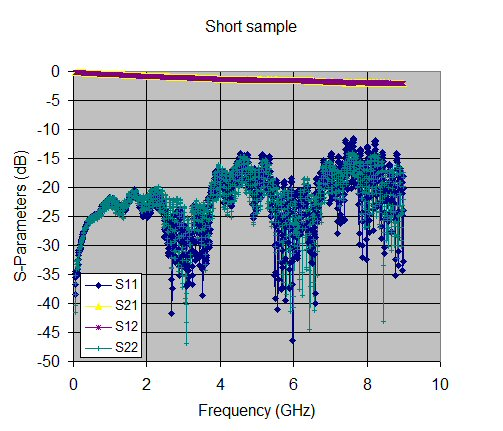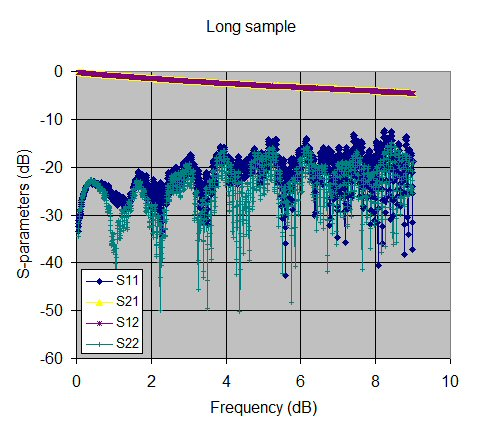First, we calculated group delay of the samples. We did this two ways, with the raw data, and by adding 11-point smoothing.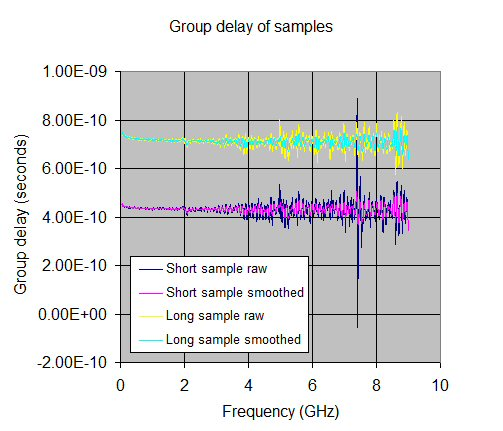From subtracting the two measurements we arrive at the group delay of the delta measurement. This is the smoothed data. Note there is still a mess of noise in it, which is going to cloud our ability to extract Dk accurately.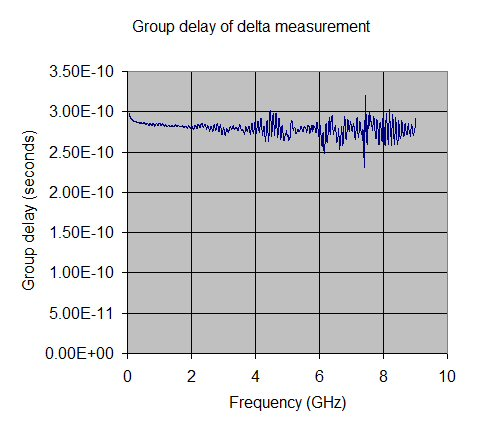From this we can calculate Keff. Then by providing a filling factor of 59.7% (we cheated and calculated FF using Agilient's ADS, more on this topic on our new filling factor page), the Dk can be extracted. The assumption we are relying on is that filling factor does not change much with the dielectric constant for a given microstrip geometry.

You can be the judge as to how well Dk is a known quantity! Let's just say it is approximately 4.3 at MHz frequencies, and somewhere around 3.8 at 9 GHz. This is slightly off from the manufacturer's supplied data (supposed to be 4.6 at MHz frequencies) but they don't tell you what the tolerance is. If it was really important to know the exact DK, we'd go back and make sure that the data is as good as possible, starting with exact measurements of the line lengths and the repeatability of the connectors used. Make sure your samples provide good return loss (-15 dB or better).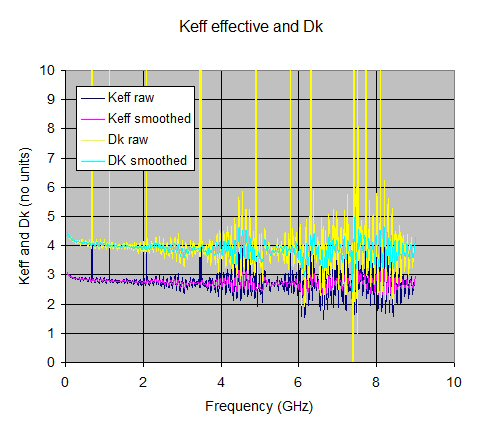Now it's time to extract the loss tangent. First we fit our model. In this case, C1 and C2 both worked out to -4. If you download our spreadsheet, you can fit to your own liking, and you will no doubt come up with slightly different numbers. Now you are getting the picture, Df measurements are in the eye of the beholder! Anyhow, we tried to fit the data closer at the lower frequencies, as the accuracy seems to crap out at higher end of the measurement.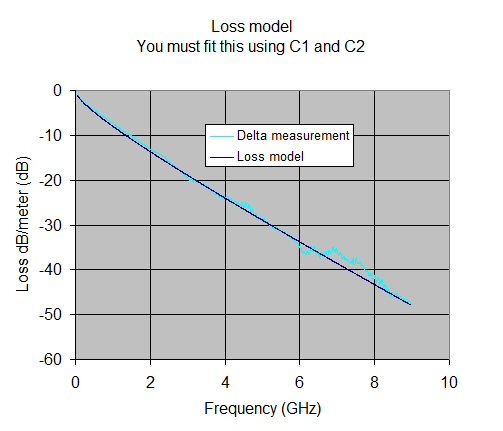From the loss model we can extract the loss tangent in two ways. Method 1 uses the modeled alphaD (which is constant versus frequency), method 2 uses the measured alphaT minus the modeled alphaM. Got that? Here the data suggest that DF is 0.027 at X-band, which is much worse that the advertised value of 0.01. As the mason said to his assistant who was bitching about having to carry concrete blocks through the mud, "what did you expect, carpeting?" In this case, what do you expect for an ultra-cheap board material?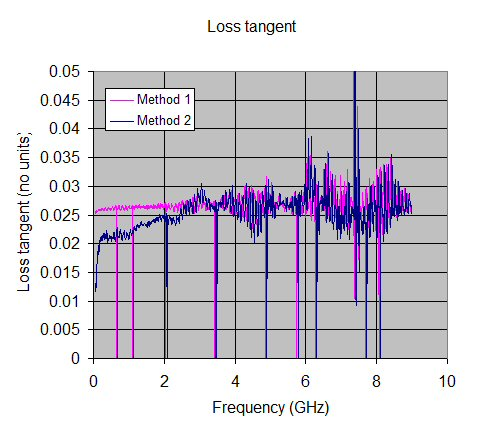In conclusion, we have shown one method of extracting Dk and Df from simple transmission line measurements. If you have a vector network analyzer, you can do this. The accuracy of the extraction depends heavily on the quality of data that is used. You must be meticulous, or the answer might be ridiculous! There's an inspirational message if there ever was one.

Later, we'll add the filling factor calculation, so that you won't need \$100,000 worth of software to get the value.

Now let's take a moment to check the analysis against an ADS model... coming soon!